# Coordinate system Questions and Answers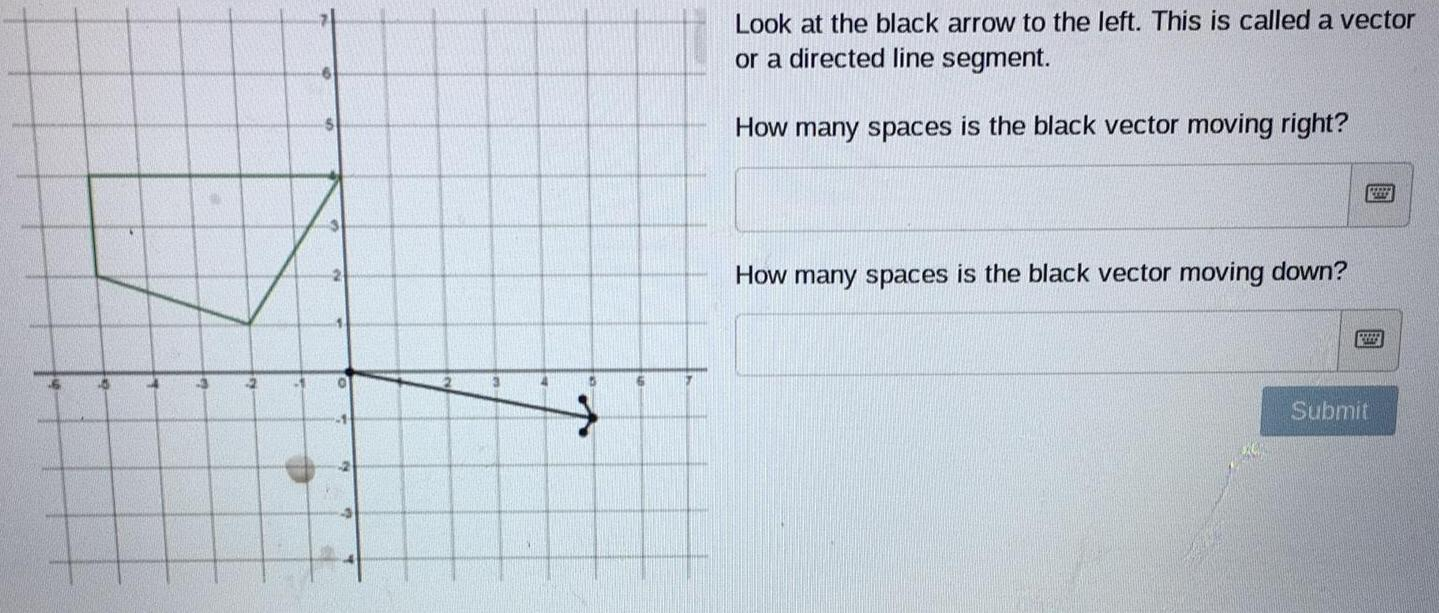Math
Coordinate system
Look at the black arrow to the left. This is called a vector or a directed line segment. How many spaces is the black vector moving right? How many spaces is the black vector moving down?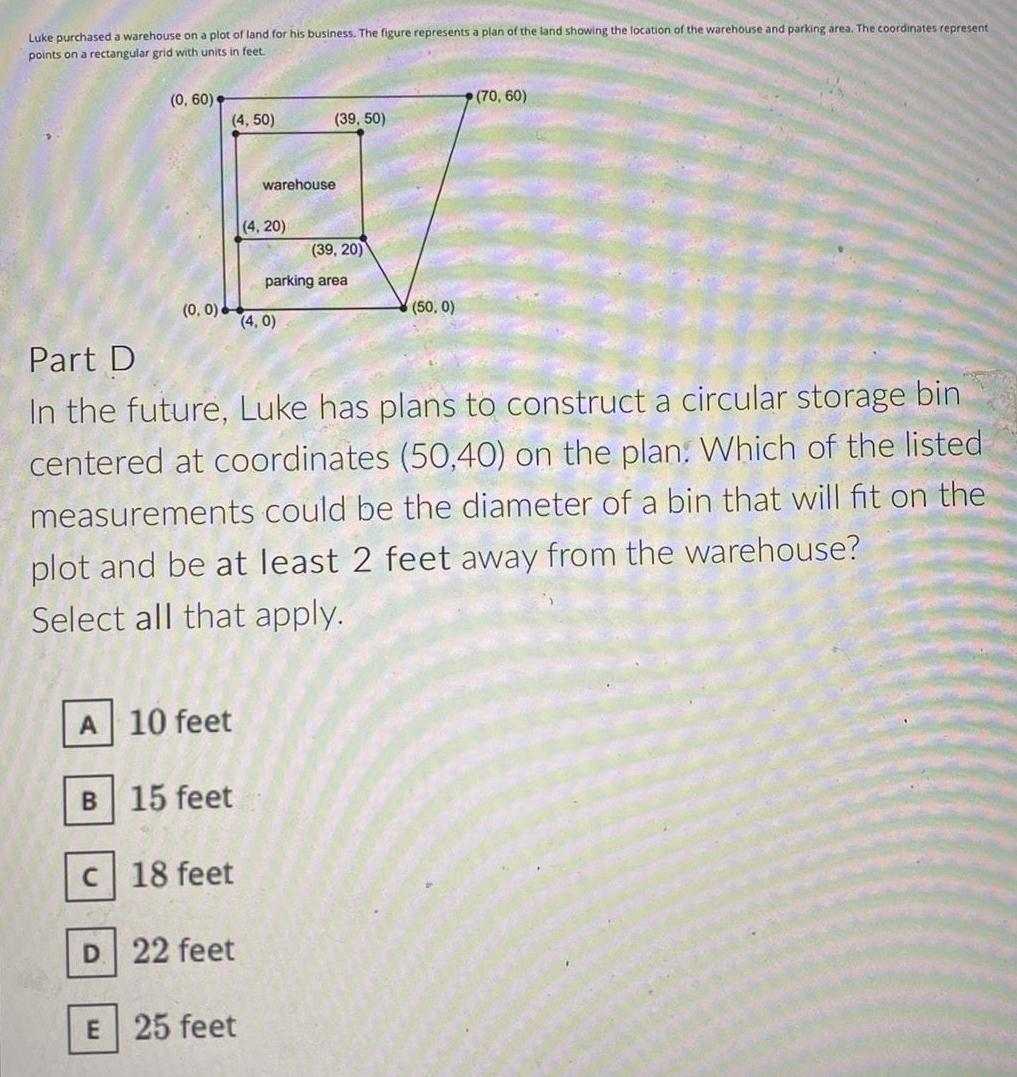Math
Coordinate system
Luke purchased a warehouse on a plot of land for his business. The figure represents a plan of the land showing the location of the warehouse and parking area. The coordinates represent points on a rectangular grid with units in feet. In the future, Luke has plans to construct a circular storage bin centered at coordinates (50,40) on the plan. Which of the listed measurements could be the diameter of a bin that will fit on the plot and be at least 2 feet away from the warehouse? Select all that apply.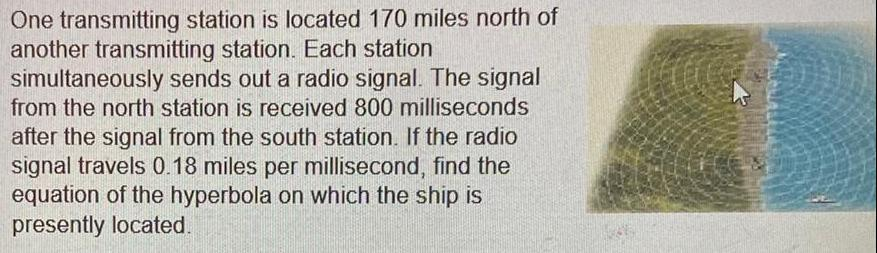Math
Coordinate system
One transmitting station is located 170 miles north of another transmitting station. Each station simultaneously sends out a radio signal. The signal from the north station is received 800 milliseconds after the signal from the south station. If the radio signal travels 0.18 miles per millisecond, find the equation of the hyperbola on which the ship is presently located.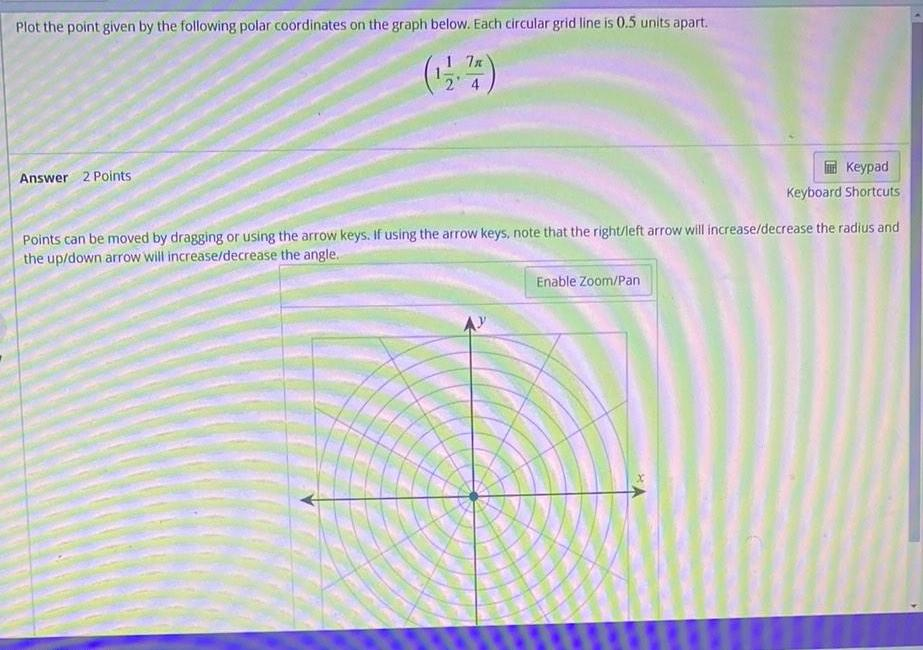Math
Coordinate system
Plot the point given by the following polar coordinates on the graph below. Each circular grid line is 0.5 units apart. Points can be moved by dragging or using the arrow keys. If using the arrow keys, note that the right/left arrow will increase/decrease the radius and the up/down arrow will increase/decrease the angle.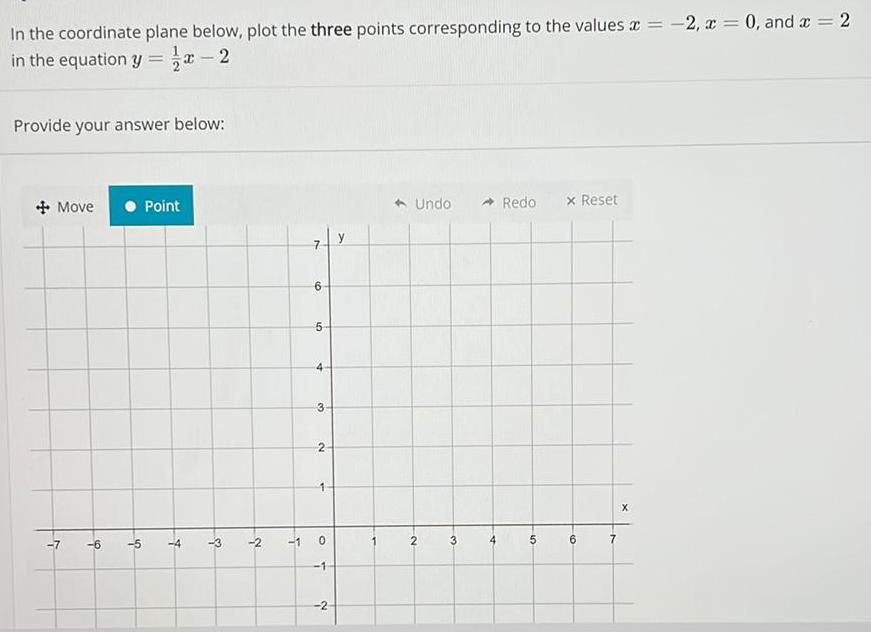Math
Coordinate system
In the coordinate plane below, plot the three points corresponding to the values x = -2, x = 0, and x = 2 in the equation y = x-2.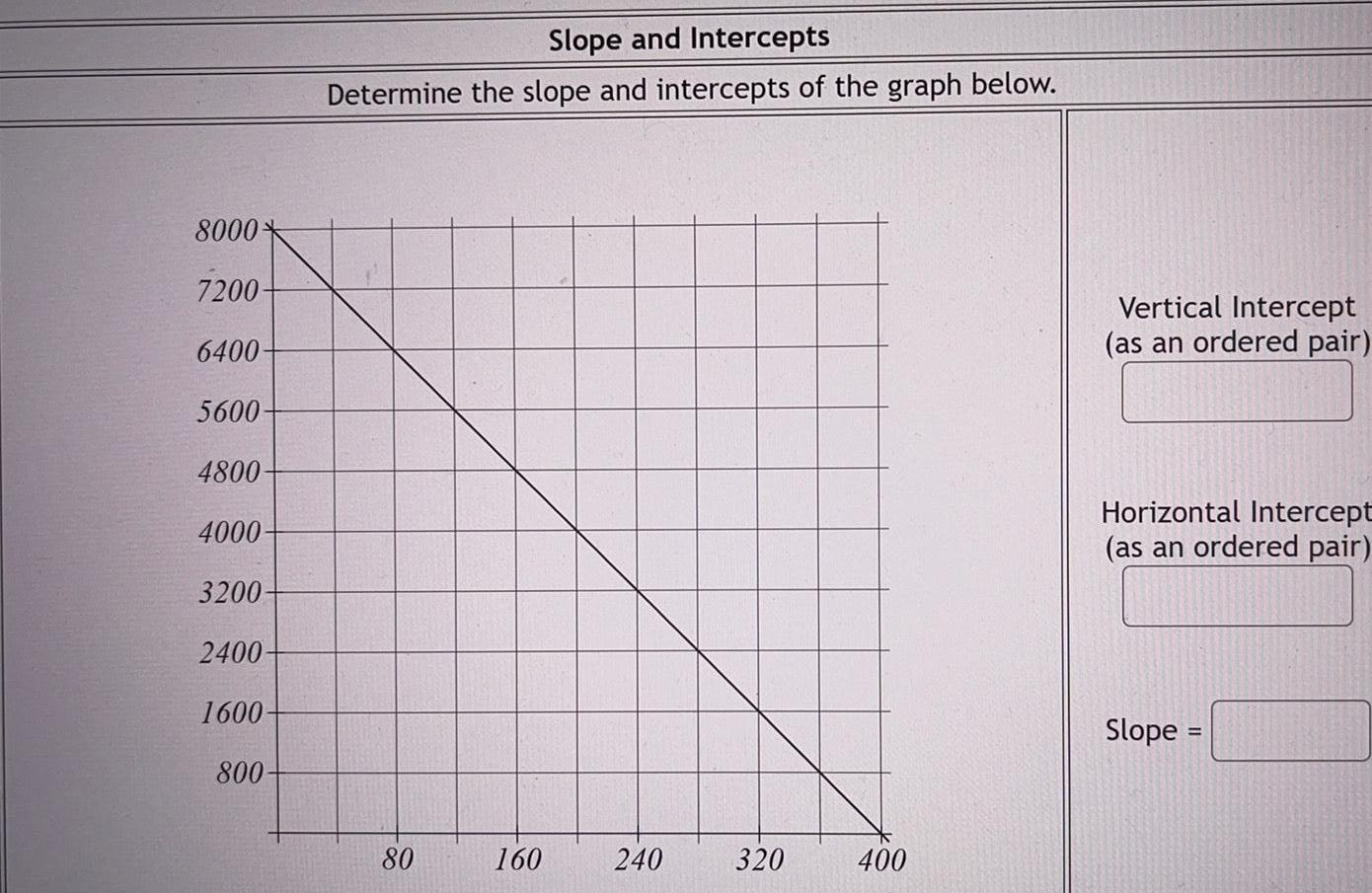Math
Coordinate system
Determine the slope and intercepts of the graph below. Vertical Intercept Horizontal Intercept
Math
Coordinate system
Consider the function: f(x) = x² + 8x - 20 The direction of the graph is like which of the following: The y-intercept is at y = The x-intercepts are at x = The vertex is at the point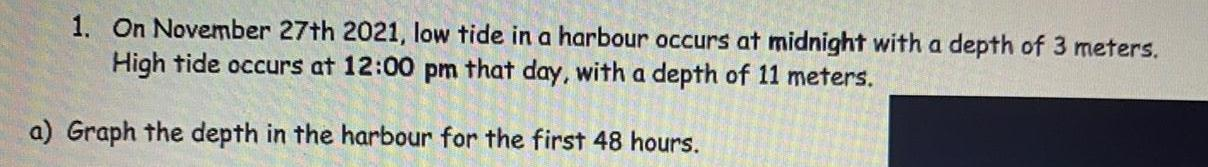Math
Coordinate system
1. On November 27th 2021, low tide in a harbour occurs at midnight with a depth of 3 meters. High tide occurs at 12:00 pm that day, with a depth of 11 meters. a) Graph the depth in the harbour for the first 48 hours.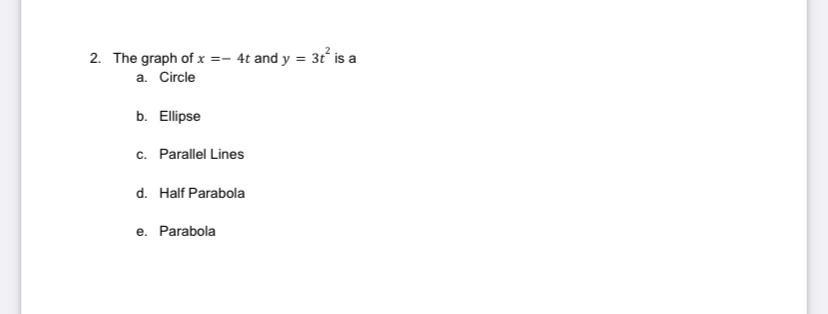Calculus
Coordinate system
The graph of x =- 4t and y = 3t^2 is a a. Circle b. Ellipse c. Parallel Lines d. Half Parabola e. Parabola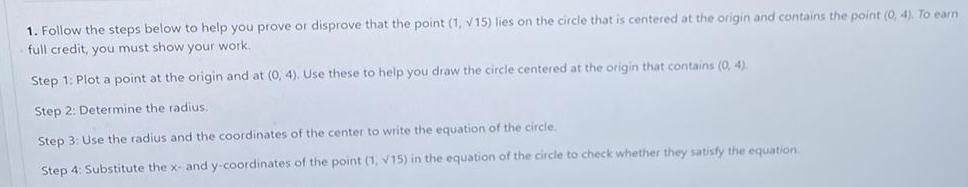Math
Coordinate system
1. Follow the steps below to help you prove or disprove that the point (1, V15) lies on the circle that is centered at the origin and contains the point (0, 4). To earn full credit, you must show your work. Step 1: Plot a point at the origin and at (0, 4). Use these to help you draw the circle centered at the origin that contains (0, 4). Step 2: Determine the radius. Step 3: Use the radius and the coordinates of the center to write the equation of the circle. Step 4: Substitute the x- and y-coordinates of the point (1, V15) in the equation of the circle to check whether they satisfy the equation.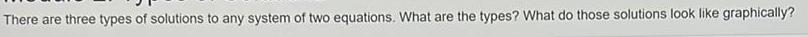Math
Coordinate system
There are three types of solutions to any system of two equations. What are the types? What do those solutions look like graphically?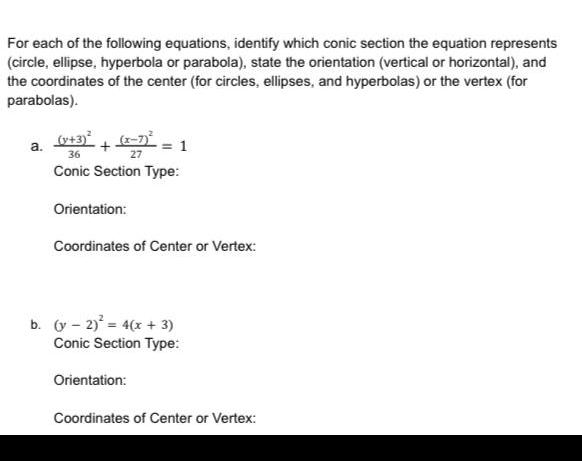Math
Coordinate system
For each of the following equations, identify which conic section the equation represents (circle, ellipse, hyperbola or parabola), state the orientation (vertical or horizontal), and the coordinates of the center (for circles, ellipses, and hyperbolas) or the vertex (for parabolas).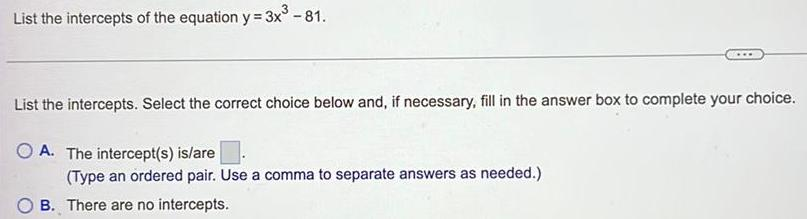Math
Coordinate system
List the intercepts of the equation y = 3x³ - 81. List the intercepts. Select the correct choice below and, if necessary, fill in the answer box to complete your choice. A. The intercept(s) is/are (Type an ordered pair. Use a comma to separate answers as needed.) B. There are no intercepts.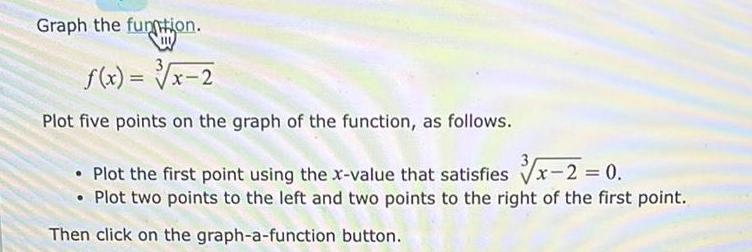Math
Coordinate system
Graph the funtion. f(x) = 3√x-2 Plot five points on the graph of the function, as follows. • Plot the first point using the x-value that satisfies √√x-2 = 0. • Plot two points to the left and two points to the right of the first point. Then click on the graph-a-function button.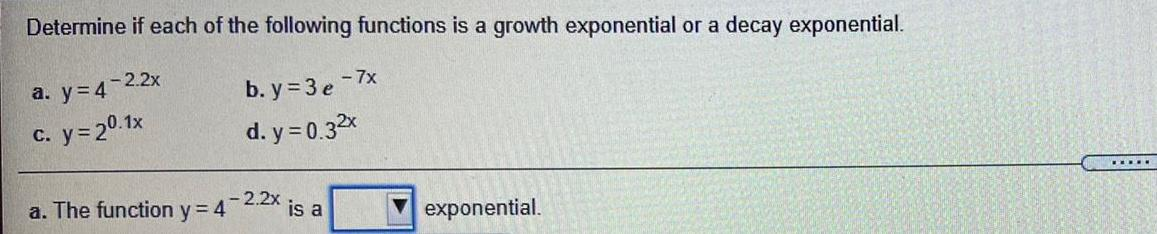Math
Coordinate system
Determine if each of the following functions is a growth exponential or a decay exponential. a.y=4-2.2x b. y=3e-7x c. y = 20.1x d.y=0.32x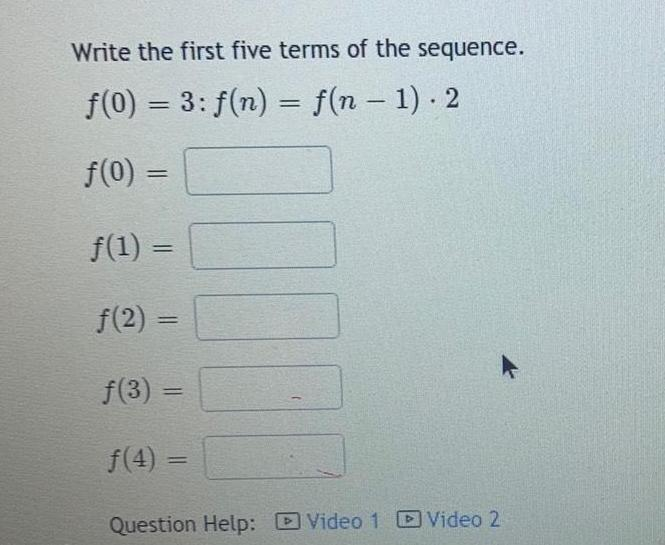Math
Coordinate system
Write the first five terms of the sequence. f(0) = 3: f(n) = f(n-1). 2 f(0) = ƒ(1) = f(2)= f(3) = f(4) = 0.00